0

# 数字图像处理之matlab实验（五）：形态学图像处理

1、结构元素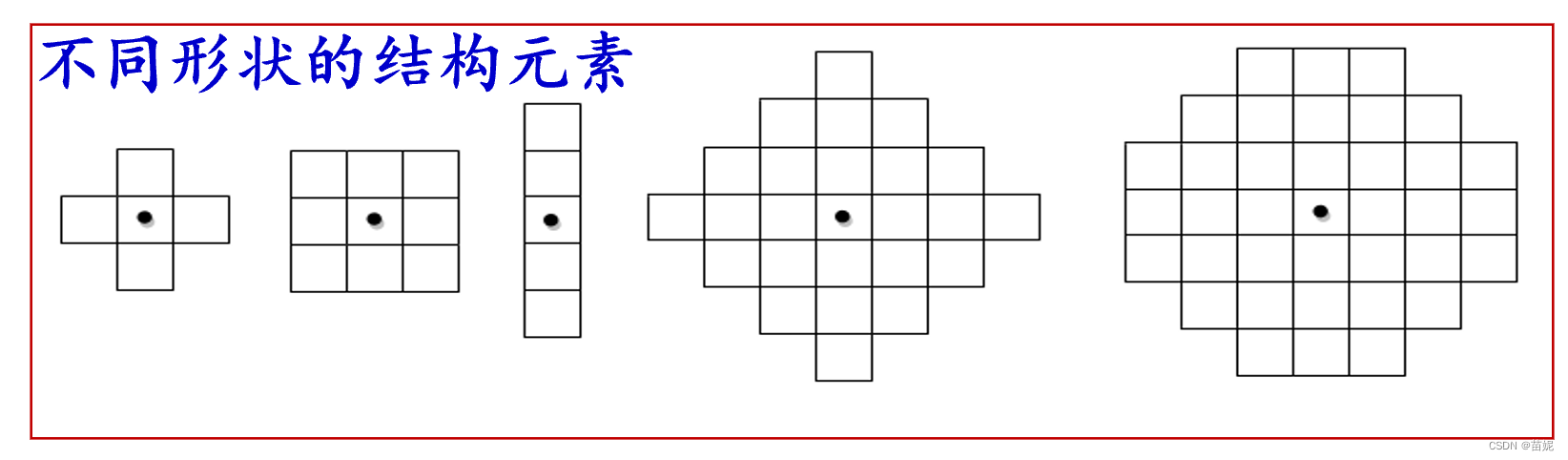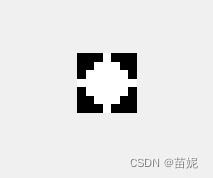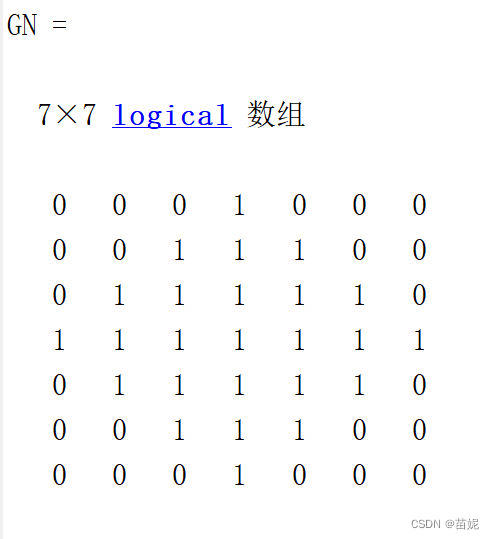``````SE = strel('diamond',3);
GN=getnhood(SE)%获取结构元素的邻域
figure,imshow(GN,[]);
``````

2、腐蚀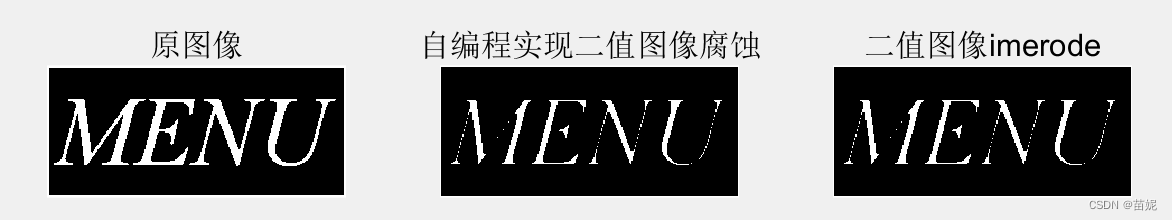``````Image=imread('menu.bmp');     %打开图像
BW=im2bw(Image);                %转换为二值图像
subplot(131),imshow(BW);title('原图像');
[h w]=size(BW);                  %获取图像尺寸
result=ones(h,w);                %定义输出图像，初始化为1
for x=2:w-1
for y=2:h-1                    %扫描图像每一点，即结构元素移动到每一个位置
for m=-1:1
for n=-1:1             %当前点周围3×3范围，即3×3结构元素所覆盖范围
if BW(y+n,x+m)==0  %该范围内有像素点为0，即该位置不能完全包含结构元素
result(y,x)=0;  %将参考点记录为背景点，即腐蚀掉
break;
end
end
end
end
end
subplot(132),imshow(result); title('自编程实现二值图像腐蚀');
SE=strel('square',3);             %创建结构元素
result=imerode(BW,SE);         %腐蚀运算
subplot(133),imshow(result); title('二值图像imerode');
``````

3、膨胀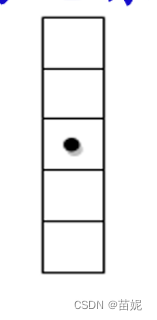``````Image=imread('menu.bmp');             %打开图像
BW=im2bw(Image);                   %转换为二值图像
subplot(131),imshow(BW),title('显示原图像');
[h w]=size(BW);                      %获取图像尺寸
result=zeros(h,w);                     %定义输出图像，初始化为0
for x=2:w-1
for y=2:h-1                      %扫描图像每一点，即结构元素移动到每一个位置
for m=-1:1
for n=-1:1               %当前点周围3×3范围，即结构元素为3×3大小
if BW(y+n,x+m)       %结构元素所覆盖3×3范围内有像素点为1，即交集不为空
result(y,x)=1;      %将参考点记录为前景点
break;
end
end
end
end
end
subplot(132),imshow(result);title('自定义实现二值图像膨胀');
SE=strel('square',3);                 %创建结构元素
result1=imdilate(BW,SE);             %膨胀运算
subplot(133),imshow(result1);title('imdilate实现二值图像膨胀');
``````

4、开运算和闭运算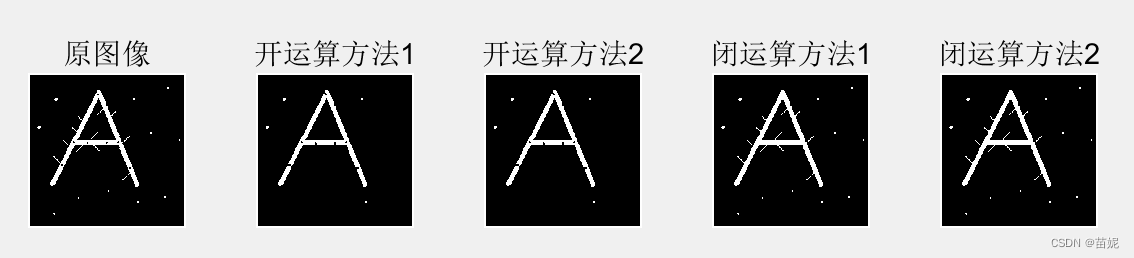``````Image=imread('A.bmp');
BW=im2bw(Image);
SE=strel('square',3);
result1=imdilate(imerode(BW,SE),SE);
result2=imopen(BW,SE);              %用3×3结构元素进行开运算
figure,imshow(result1);title('开运算方法1');
figure,imshow(result2);title('开运算方法2');
result3=imerode(imdilate(BW,SE),SE);
result4=imclose(BW,SE);             %用3×3结构元素进行闭运算
figure,imshow(result3);title('闭运算方法1');
figure,imshow(result4);title('闭运算方法2');
``````

5、形态学滤波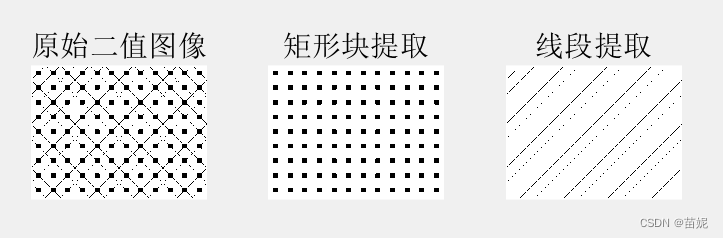``````Image=imread('pattern.jpg');
Th=graythresh(Image);
OriginBW=im2bw(Image,Th);
subplot(131);imshow(OriginBW);title('原始二值图像');
imwrite(OriginBW,'pattern1.bmp');
BW1=1-OriginBW;
se=strel('square',3);%结构元素为边长为3的正方形
BW2=1-imopen(BW1,se);
subplot(132);imshow(BW2);title('矩形块提取');
imwrite(BW2,'rectang1.bmp');
se45=strel('line',25,45);%结构元素为角度为45的线，长度为25个像素
BW3=1-imopen(BW1,se45);
subplot(133);imshow(BW3);title('线段提取');
imwrite(BW3,'line1.bmp');
``````

6、平滑处理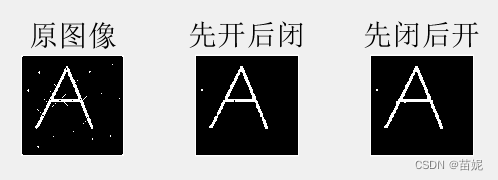``````Image=imread('A.bmp');
BW=im2bw(Image);
subplot(131);imshow(BW);title('原图像');
SE=strel('square',4);
result1=imclose(imopen(BW,SE),SE);               %用3×3结构元素先开后闭
subplot(132);imshow(result1);title('先开后闭');
result2=imopen(imclose(BW,SE),SE);               %先闭后开
subplot(133);imshow(result2);title('先闭后开');
``````

7、击中与不击中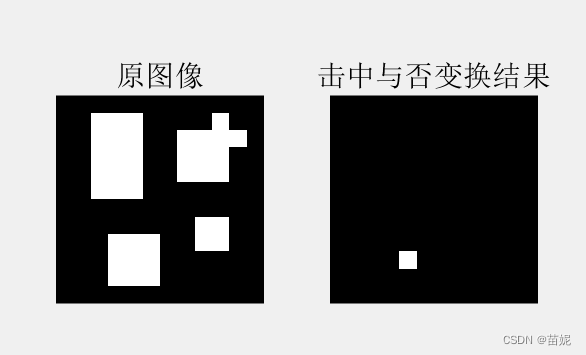``````Image=zeros(12,12);   %定义目标图像Image
Image(2:6,3:5)=1;
Image(9:11,4:6)=1;
Image(3:5,8:10)=1;
Image(8:9,9:10)=1;
Image(2,10)=1;
Image(3,11)=1;
SE1=[0 0 0 0 0    %定义结构元素SE1
0 1 1 1 0
0 1 1 1 0
0 1 1 1 0
0 0 0 0 0];
SE2=[1 1 1 1 1    %定义结构元素SE2
1 0 0 0 1
1 0 0 0 1
1 0 0 0 1
1 1 1 1 1];
subplot(121),imshow(Image); title('原图像');
result1=imerode(Image,SE1); %结构元素SE1探测图像内部，结果为result1
Image1=~Image;  %目标图像Image求补
result2=imerode(Image1,SE2); %结构元素SE2检测图像外部，结果为result2
result=result1 & result2; %求出击中与否变换的结果result
subplot(122),imshow(result); title('击中与否变换结果');
``````

1、腐蚀和膨胀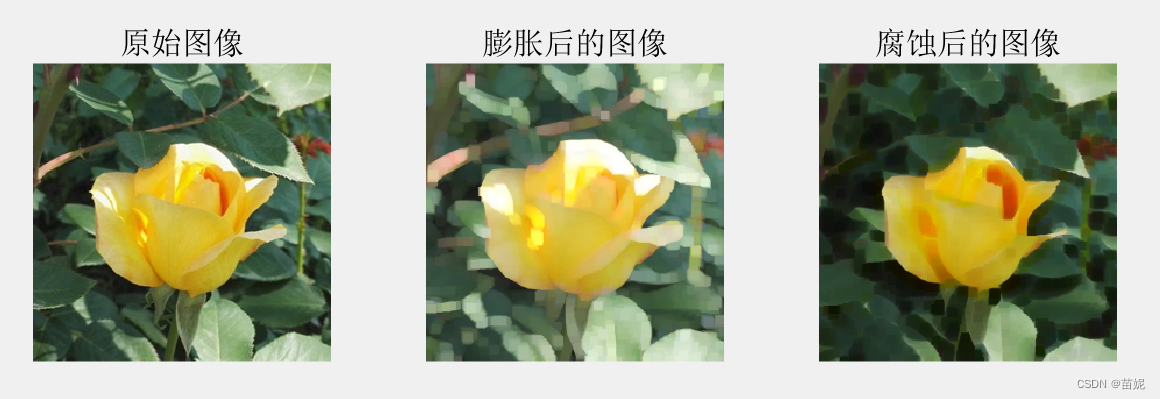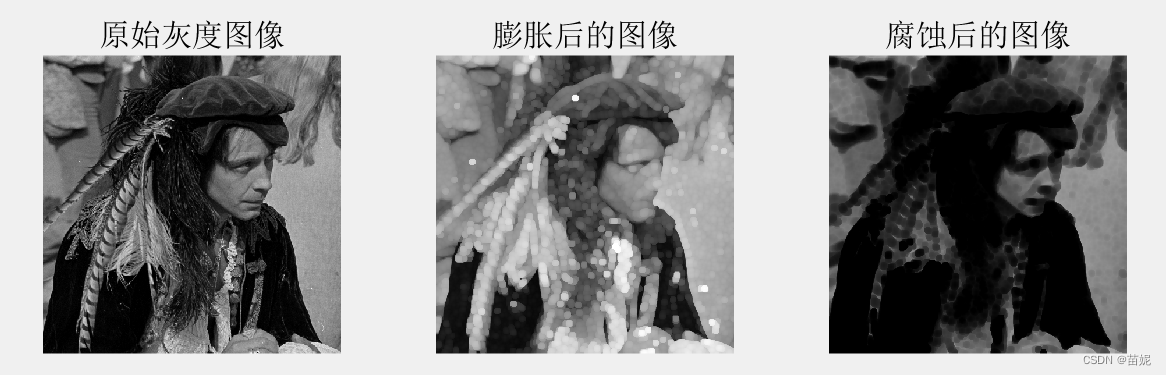``````Image=(imread('maleman.gif'));
se=strel('ball',5,5);%选取球形结构元素
result1=imdilate(Image,se);%膨胀灰度图像
result2=imerode(Image,se); %腐蚀灰度图像
imshow(Image);title('原始灰度图像');
figure,imshow(result1);title('膨胀后的图像');
figure,imshow(result2);title('腐蚀后的图像');
imwrite(result1,'ym1.bmp');
imwrite(result2,'ym2.bmp');
``````

### “数字图像处理之matlab实验（五）：形态学图像处理”的评论:

##### 关于作者##### overfit同步小助手